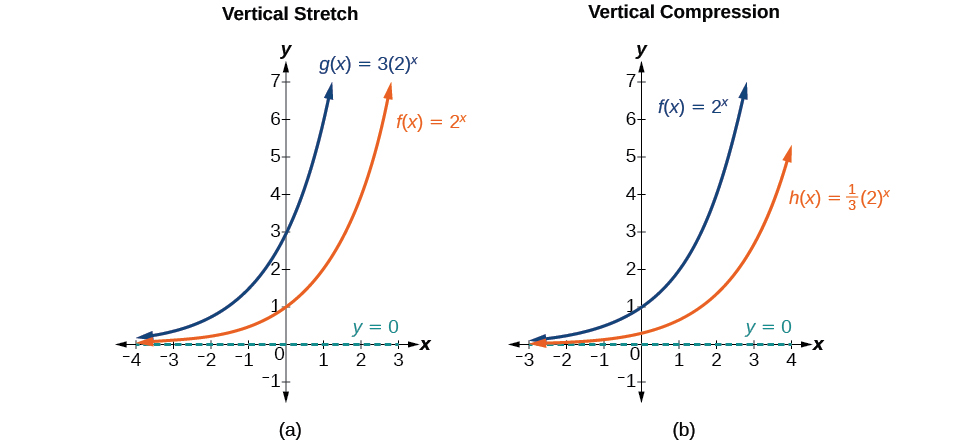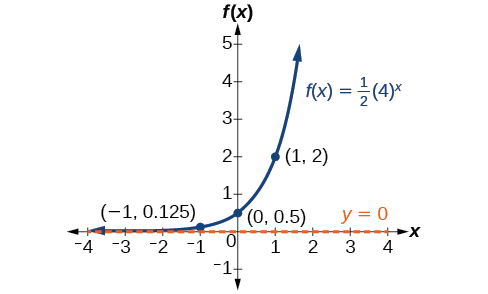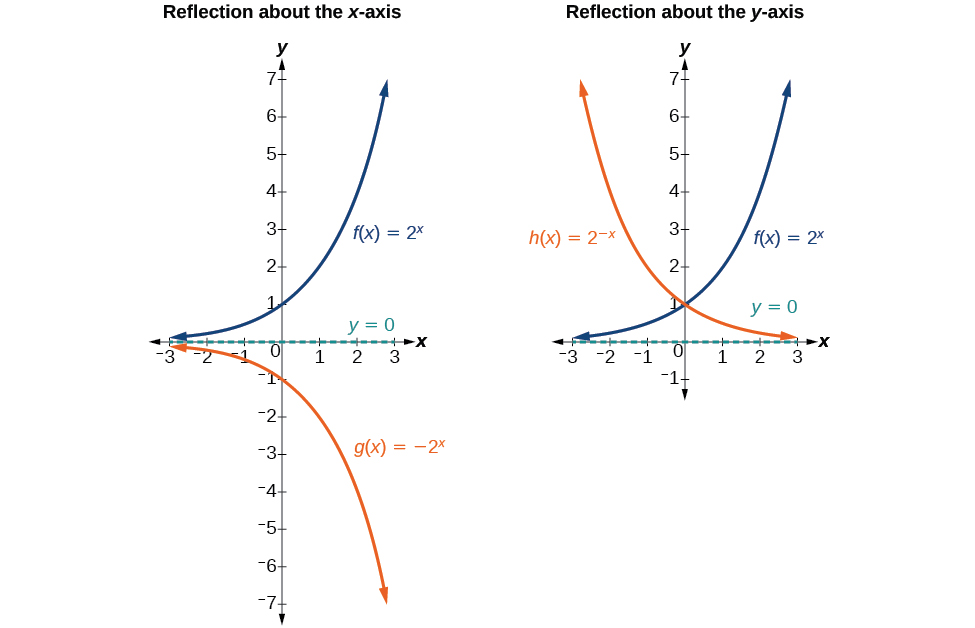# 6.2 Graphs of exponential functions  (Page 4/6)

 Page 4 / 6(a)   g ( x ) = 3 ( 2 ) x   stretches the graph of   f ( x ) = 2 x   vertically by a factor of   3.   (b)   h ( x ) = 1 3 ( 2 ) x   compresses the graph of   f ( x ) = 2 x   vertically by a factor of   1 3 .

## Stretches and compressions of the parent function f ( x ) = b x

For any factor $\text{\hspace{0.17em}}a>0,$ the function $\text{\hspace{0.17em}}f\left(x\right)=a{\left(b\right)}^{x}$

• is stretched vertically by a factor of $\text{\hspace{0.17em}}a\text{\hspace{0.17em}}$ if $\text{\hspace{0.17em}}|a|>1.$
• is compressed vertically by a factor of $\text{\hspace{0.17em}}a\text{\hspace{0.17em}}$ if $\text{\hspace{0.17em}}|a|<1.$
• has a y -intercept of $\text{\hspace{0.17em}}\left(0,a\right).$
• has a horizontal asymptote at $\text{\hspace{0.17em}}y=0,$ a range of $\text{\hspace{0.17em}}\left(0,\infty \right),$ and a domain of $\text{\hspace{0.17em}}\left(-\infty ,\infty \right),$ which are unchanged from the parent function.

## Graphing the stretch of an exponential function

Sketch a graph of $\text{\hspace{0.17em}}f\left(x\right)=4{\left(\frac{1}{2}\right)}^{x}.\text{\hspace{0.17em}}$ State the domain, range, and asymptote.

Before graphing, identify the behavior and key points on the graph.

• Since $\text{\hspace{0.17em}}b=\frac{1}{2}\text{\hspace{0.17em}}$ is between zero and one, the left tail of the graph will increase without bound as $\text{\hspace{0.17em}}x\text{\hspace{0.17em}}$ decreases, and the right tail will approach the x -axis as $\text{\hspace{0.17em}}x\text{\hspace{0.17em}}$ increases.
• Since $\text{\hspace{0.17em}}a=4,$ the graph of $\text{\hspace{0.17em}}f\left(x\right)={\left(\frac{1}{2}\right)}^{x}\text{\hspace{0.17em}}$ will be stretched by a factor of $\text{\hspace{0.17em}}4.$
• Create a table of points as shown in [link] .  $x$ $-3$ $-2$ $-1$ $0$ $1$ $2$ $3$ $f\left(x\right)=4\left(\frac{1}{2}{\right)}^{x}$ $32$ $16$ $8$ $4$ $2$ $1$ $0.5$
• Plot the y- intercept, $\text{\hspace{0.17em}}\left(0,4\right),$ along with two other points. We can use $\text{\hspace{0.17em}}\left(-1,8\right)\text{\hspace{0.17em}}$ and $\text{\hspace{0.17em}}\left(1,2\right).$

Draw a smooth curve connecting the points, as shown in [link] .

The domain is $\text{\hspace{0.17em}}\left(-\infty ,\infty \right);\text{\hspace{0.17em}}$ the range is $\text{\hspace{0.17em}}\left(0,\infty \right);\text{\hspace{0.17em}}$ the horizontal asymptote is $\text{\hspace{0.17em}}y=0.$

Sketch the graph of $\text{\hspace{0.17em}}f\left(x\right)=\frac{1}{2}{\left(4\right)}^{x}.\text{\hspace{0.17em}}$ State the domain, range, and asymptote.

The domain is $\text{\hspace{0.17em}}\left(-\infty ,\infty \right);\text{\hspace{0.17em}}$ the range is $\text{\hspace{0.17em}}\left(0,\infty \right);\text{\hspace{0.17em}}$ the horizontal asymptote is $\text{\hspace{0.17em}}y=0.\text{\hspace{0.17em}}$## Graphing reflections

In addition to shifting, compressing, and stretching a graph, we can also reflect it about the x -axis or the y -axis. When we multiply the parent function $\text{\hspace{0.17em}}f\left(x\right)={b}^{x}\text{\hspace{0.17em}}$ by $\text{\hspace{0.17em}}-1,$ we get a reflection about the x -axis. When we multiply the input by $\text{\hspace{0.17em}}-1,$ we get a reflection about the y -axis. For example, if we begin by graphing the parent function $\text{\hspace{0.17em}}f\left(x\right)={2}^{x},$ we can then graph the two reflections alongside it. The reflection about the x -axis, $\text{\hspace{0.17em}}g\left(x\right)={-2}^{x},$ is shown on the left side of [link] , and the reflection about the y -axis $\text{\hspace{0.17em}}h\left(x\right)={2}^{-x},$ is shown on the right side of [link] .(a)   g ( x ) = − 2 x   reflects the graph of   f ( x ) = 2 x   about the x-axis. (b)   g ( x ) = 2 − x   reflects the graph of   f ( x ) = 2 x   about the y -axis.

## Reflections of the parent function f ( x ) = b x

The function $\text{\hspace{0.17em}}f\left(x\right)=-{b}^{x}$

• reflects the parent function $\text{\hspace{0.17em}}f\left(x\right)={b}^{x}\text{\hspace{0.17em}}$ about the x -axis.
• has a y -intercept of $\text{\hspace{0.17em}}\left(0,-1\right).$
• has a range of $\text{\hspace{0.17em}}\left(-\infty ,0\right)$
• has a horizontal asymptote at $\text{\hspace{0.17em}}y=0\text{\hspace{0.17em}}$ and domain of $\text{\hspace{0.17em}}\left(-\infty ,\infty \right),$ which are unchanged from the parent function.

The function $\text{\hspace{0.17em}}f\left(x\right)={b}^{-x}$

• reflects the parent function $\text{\hspace{0.17em}}f\left(x\right)={b}^{x}\text{\hspace{0.17em}}$ about the y -axis.
• has a y -intercept of $\text{\hspace{0.17em}}\left(0,1\right),$ a horizontal asymptote at $\text{\hspace{0.17em}}y=0,$ a range of $\text{\hspace{0.17em}}\left(0,\infty \right),$ and a domain of $\text{\hspace{0.17em}}\left(-\infty ,\infty \right),$ which are unchanged from the parent function.

## Writing and graphing the reflection of an exponential function

Find and graph the equation for a function, $\text{\hspace{0.17em}}g\left(x\right),$ that reflects $\text{\hspace{0.17em}}f\left(x\right)={\left(\frac{1}{4}\right)}^{x}\text{\hspace{0.17em}}$ about the x -axis. State its domain, range, and asymptote.

Since we want to reflect the parent function $\text{\hspace{0.17em}}f\left(x\right)={\left(\frac{1}{4}\right)}^{x}\text{\hspace{0.17em}}$ about the x- axis, we multiply $\text{\hspace{0.17em}}f\left(x\right)\text{\hspace{0.17em}}$ by $\text{\hspace{0.17em}}-1\text{\hspace{0.17em}}$ to get, $\text{\hspace{0.17em}}g\left(x\right)=-{\left(\frac{1}{4}\right)}^{x}.\text{\hspace{0.17em}}$ Next we create a table of points as in [link] .

 $x$ $-3$ $-2$ $-1$ $0$ $1$ $2$ $3$ $g\left(x\right)=-\left(\frac{1}{4}{\right)}^{x}$ $-64$ $-16$ $-4$ $-1$ $-0.25$ $-0.0625$ $-0.0156$

Plot the y- intercept, $\text{\hspace{0.17em}}\left(0,-1\right),$ along with two other points. We can use $\text{\hspace{0.17em}}\left(-1,-4\right)\text{\hspace{0.17em}}$ and $\text{\hspace{0.17em}}\left(1,-0.25\right).$

Draw a smooth curve connecting the points:

The domain is $\text{\hspace{0.17em}}\left(-\infty ,\infty \right);\text{\hspace{0.17em}}$ the range is $\text{\hspace{0.17em}}\left(-\infty ,0\right);\text{\hspace{0.17em}}$ the horizontal asymptote is $\text{\hspace{0.17em}}y=0.$

use the y -intercept and slope to sketch the graph of the equation y=6x
how do we prove the quadratic formular
hello, if you have a question about Algebra 2. I may be able to help. I am an Algebra 2 Teacher
thank you help me with how to prove the quadratic equation
Seidu
may God blessed u for that. Please I want u to help me in sets.
Opoku
what is math number
4
Trista
x-2y+3z=-3 2x-y+z=7 -x+3y-z=6
Need help solving this problem (2/7)^-2
x+2y-z=7
Sidiki
what is the coefficient of -4×
-1
Shedrak
the operation * is x * y =x + y/ 1+(x × y) show if the operation is commutative if x × y is not equal to -1
An investment account was opened with an initial deposit of \$9,600 and earns 7.4% interest, compounded continuously. How much will the account be worth after 15 years?
lim x to infinity e^1-e^-1/log(1+x)
given eccentricity and a point find the equiation
12, 17, 22.... 25th term
12, 17, 22.... 25th term
Akash
College algebra is really hard?
Absolutely, for me. My problems with math started in First grade...involving a nun Sister Anastasia, bad vision, talking & getting expelled from Catholic school. When it comes to math I just can't focus and all I can hear is our family silverware banging and clanging on the pink Formica table.
Carole
I'm 13 and I understand it great
AJ
I am 1 year old but I can do it! 1+1=2 proof very hard for me though.
Atone
Not really they are just easy concepts which can be understood if you have great basics. I am 14 I understood them easily.
Vedant
hi vedant can u help me with some assignments
Solomon
find the 15th term of the geometric sequince whose first is 18 and last term of 387
I know this work
salma
The given of f(x=x-2. then what is the value of this f(3) 5f(x+1)
hmm well what is the answer
Abhi
If f(x) = x-2 then, f(3) when 5f(x+1) 5((3-2)+1) 5(1+1) 5(2) 10
AugustineByByByBy RhodesByBy Rhodes